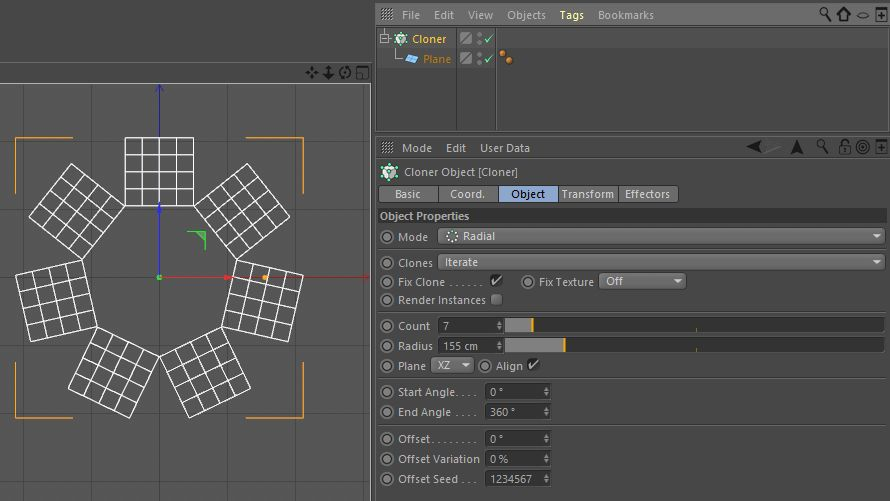# SOLVEDFormula to calculate cloner radius

Hi,
I'm searching the formula to automatically calculate the Cloner object radius depending on the number of clones (Count) and the Plain object width to obtain a perfect circle of objects without any objects spacing or overlap. see screenshot below:Thanks.

Hi mfersaoui, thanks for reaching out us.

With regard to your question given the length of the side l and the number of sides n you can calculate the apothem of a regular polygon using the following formula:

` apothem = l / (2 * tan ( 180 / n ) )`

Be aware that being the objects distributed with regard to their origin, the half of the height of the polygon should also be considered.

Be aware that you're intested in the apothem value and not in the real radius cause the Cloner actually use this value.

Cheers, Riccardo

Hi mfersaoui, thanks for reaching out us.

With regard to your question given the length of the side l and the number of sides n you can calculate the apothem of a regular polygon using the following formula:

` apothem = l / (2 * tan ( 180 / n ) )`

Be aware that being the objects distributed with regard to their origin, the half of the height of the polygon should also be considered.

Be aware that you're intested in the apothem value and not in the real radius cause the Cloner actually use this value.

Cheers, Riccardo

@r_gigante

Hi Riccardo, thank you for your detailed response. That allowed me to find the exact formula.

``````l / (2 * tan((180 / n) * 3.14159 / 180))
``````

Regards, Mustapha